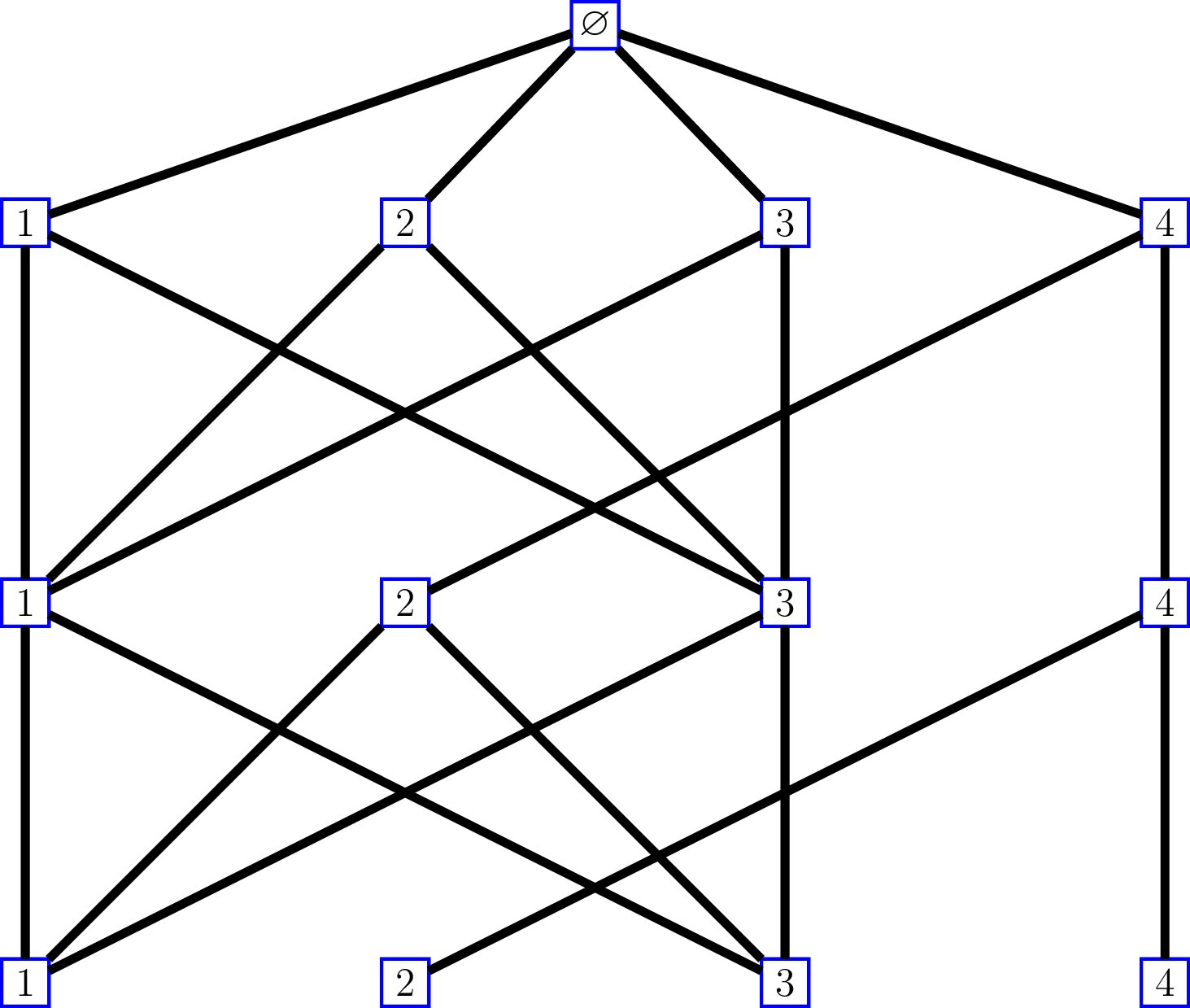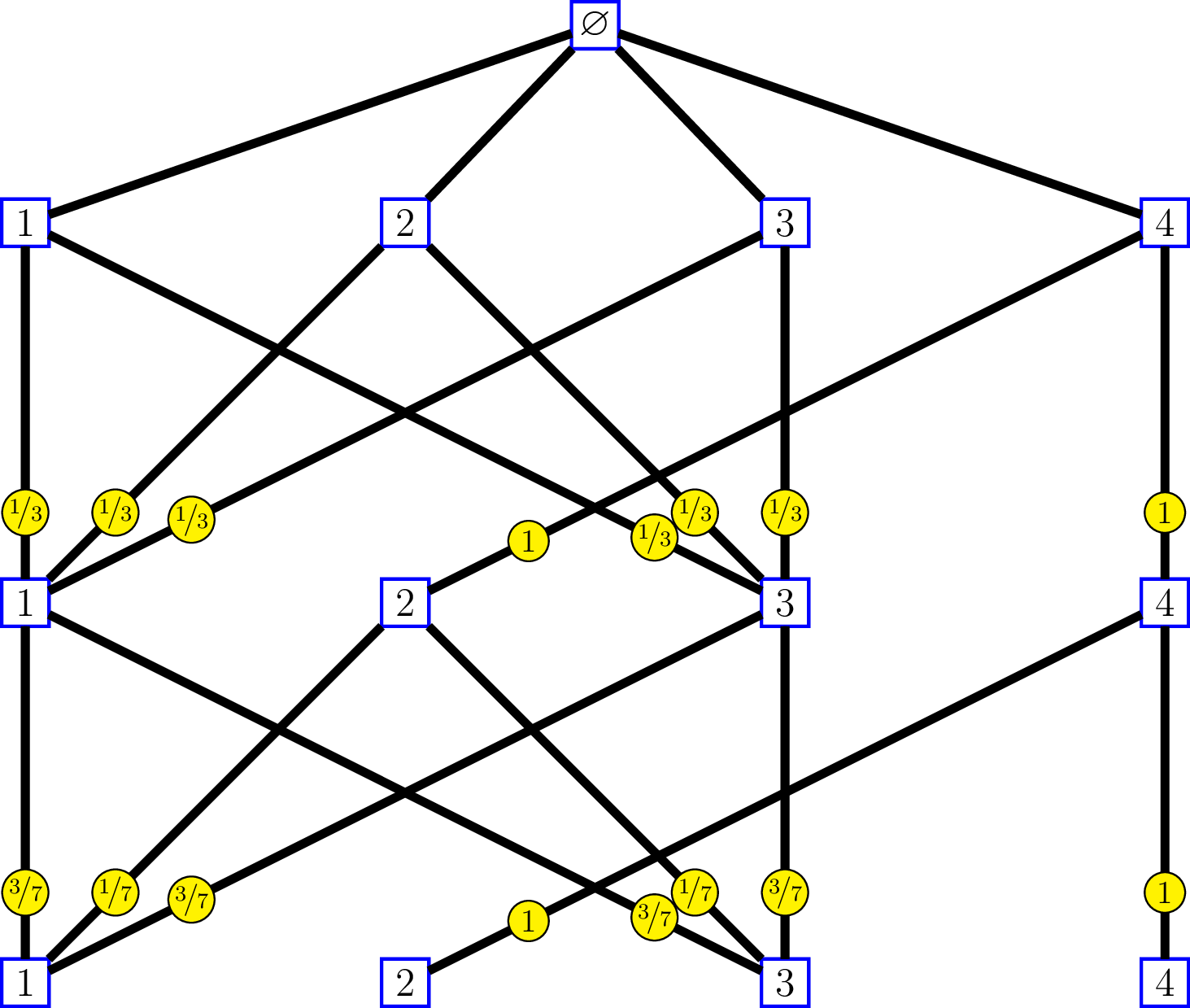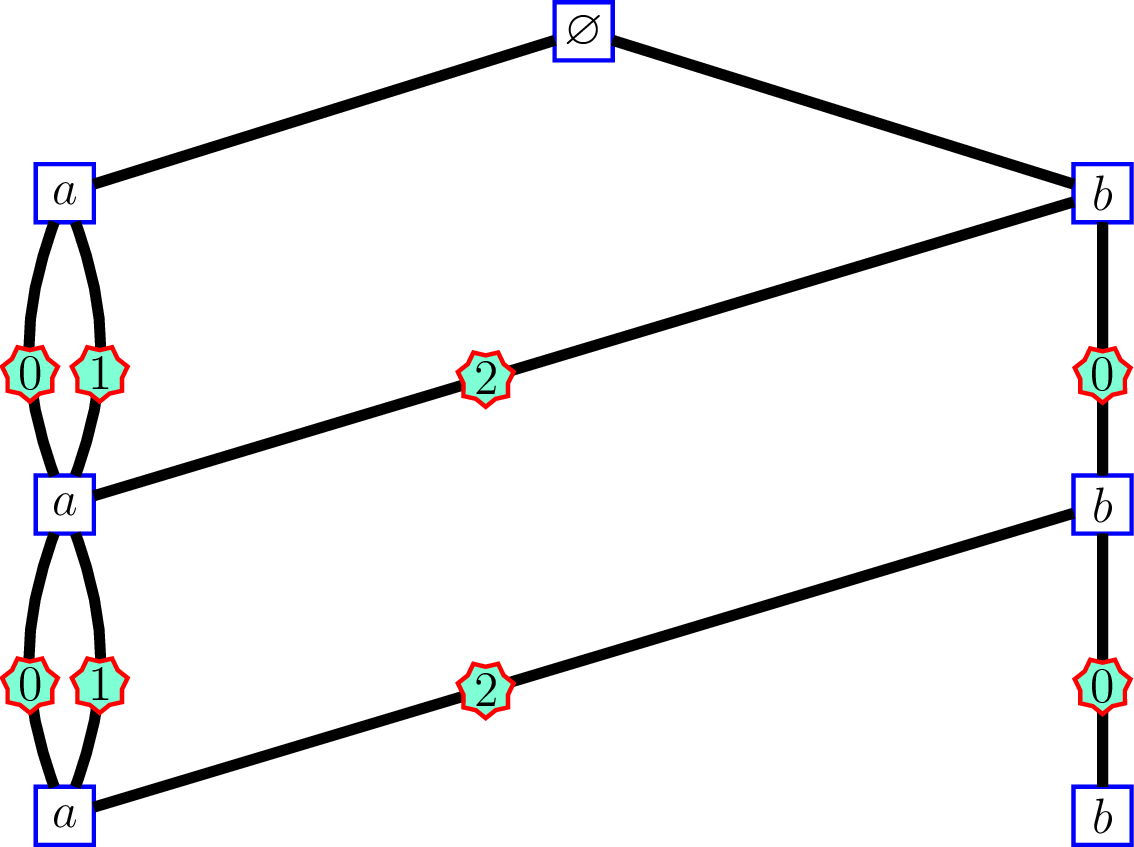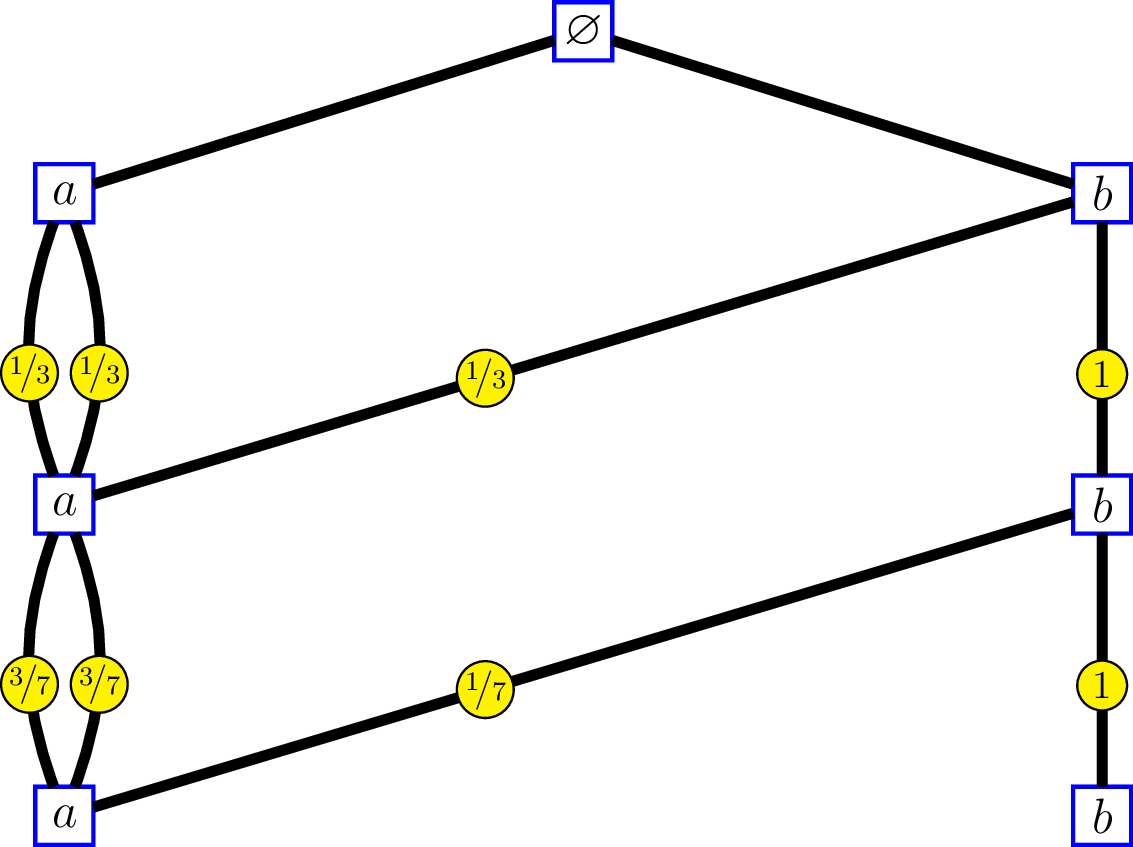--- title: "Bratteli graph: from simple to multiple edges" author: "Stéphane Laurent" date: "22 février 2016" output: html_document --- In my research in mathematics, I deal with the filtration generated by a "random walk" on a Bratteli graph, whose distribution is a *central measure*, that is to say the transition distributions from a level to the above level are the [central kernels](http://stla.github.io/stlapblog/posts/IntrinsicDistances.html). In some situations it is possible to replace the graph with another graph having multiple edges and less vertices. Consider for instance the following Bratteli graph:whose incidence matrices are $$M_0=\begin{pmatrix} 1 & 1 & 1 & 1 \end{pmatrix}, \, \text{and } M_n = \begin{pmatrix} 1 & 0 & 1 & 0 \\ 1 & 0 & 1 & 0 \\ 1 & 0 & 1 & 0 \\ 0 & 1 & 0 & 1 \end{pmatrix}\, \text{for } n\geq 1.$$ The labels on the edges of the graph at the right side show the [intrinsic kernels](http://stla.github.io/stlapblog/posts/IntrinsicDistances.html). We'll come back later to that. Observe that the first and thirds columns of $M_n$ are the same: each of the two vertices $1$ and $3$ at level $n$ are connected to vertices $1$, $2$ and $3$ at level $n-1$. The second and fourth columns are the same as well: each of the two vertices $2$ and $4$ at level $n$ are connected to vertex $4$ at level $n-1$. Identify an infinite path of this graph by a sequence of vertices $(v_1, v_2, \ldots)$, without including the root vertex which is useless. On the other hand, consider the following Bratteli graph:whose incidence matrices are $$M_0 = \begin{pmatrix} 3 & 1 \end{pmatrix}, \, \text{and } M_n = \begin{pmatrix} 2 & 0 \\ 1 & 1 \end{pmatrix}\, \text{for } n\geq 1.$$ The edges of the graph at left side are labelled by the "[order labels](http://stla.github.io/stlapblog/posts/OrderedBratteli.html)". For this graph, identify an infinite path by the sequence $\bigl((v'_1, e_1), (v'_2, e_2), \ldots, \bigr)$ where $v'_n$ is the vertex at level $n$ and $e_n$ is the label of the edge connecting $v'_n$ and $v'_{n+1}$. Then there is a correspondance between the set of sequences identifying the paths of the first graph and the set of sequences identifying the paths of the second. At each level $n$: - vertex $v_n=\,$$1 on the first graph corresponds to (v'_n,e_n)=(a, 0) - vertex v_n=\,$$2$ on the first graph corresponds to $(v'_n,e_n)=(b, 2)$ - vertex $v_n=\,$$3 on the first graph corresponds to (v'_n,e_n)=(a, 1) - vertex v_n=\,$$4$ on the first graph corresponds to $(v'_n,e_n)=(b, 0)$ At the right side, the labels on the edges show the intrinsic kernels. Consider a "random walk" ${(V_n)}_{n \geq 1}$ on the first graph, whose transitions from level $n$ to level $n-1$ are given by these kernels: $$\begin{pmatrix} 1/3 & 1/3 & 1/3 & 0 \\ 0 & 0 & 0 & 1 \\ 1/3 & 1/3 & 1/3 & 0 \\ 0 & 0 & 0 & 1 \end{pmatrix}, \begin{pmatrix} 3/7 & 1/7 & 3/7 & 0 \\ 0 & 0 & 0 & 1 \\ 3/7 & 1/7 & 3/7 & 0 \\ 0 & 0 & 0 & 1 \end{pmatrix}, \ldots.$$ and send ${(V_n)}_{n \geq 1}$ to a "random walk" ${(V'_n, \epsilon_n)}_{n \geq 1}$ on the second graph by the correspondance $v_n \mapsto (v'_n,e_n)$ previously seen. Therefore the reversed filtrations generated by the two random walks are isomorphic, and the transitions of ${(V'_n, \epsilon_n)}_{n \geq 1}$ from level $n$ to level $n-1$ are given by the intrinsic kernels.